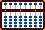###PREDETERMINING THE UNIT ROD

The Bead Unbaffled - An Abacus Manual

When I first began to solve problems of multiplication and division on my soroban I found that decimal numbers could be confusing. The following is a method that allows for placing both multiplicand and dividend onto the soroban in such a way that the unit and decimal numbers in both product and quotient fall naturally on predetermined rods. In other words you always know where your unit rod is. Take the example 0.00008 ÷ 0.002 = 0.04   Because you've already predetermined the decimal place, what looks like a rather complicated decimal problem involving a lot of zeros becomes a much more manageable 8 ÷ 2 = 4

This method is a variation on one taught to me by Edvaldo Siqueira of Rio de Janeiro, Brazil. The method was developed by Professor Fukutaro Kato, a Japanese soroban teacher living in Brazil in the 1960's and was published in the Professor's book, *SOROBAN pelo Método Moderno*. (*SOROBAN by the Modern Method*)

As some of the following examples will reveal, the method works particularly well and helps to simplify problems having complicated decimal numbers.

### Counting Digits

Where digits are whole numbers or mixed decimal numbers, count only the whole number before the decimal. Consider the result positive.

Examples:

```0.253.......count  0 digits.
2.703.......count +1 digit.
56.0092.....count +2 digits.
459.38......count +3 digits.
1500........count +4 digits, and so on.```

Where digits are pure decimal numbers, count only the zeros that immediately follow the decimal. Consider the result negative.

Examples:

```0.40077.....count  0 digits.
0.02030.....count -1 digit.
0.0092......count -2 digits.
0.00057.....count -3 digits, and so on.```

### Setting Problems On The Soroban

Multiplication:

Soroban showing rods A through K with *F* acting as the unit rod;

----A----B----C----D----E---*F*---G----H----I----J----K----
...+5...+4...+3...+2...+1....0...-1...-2...-3...-4...-5....

Formula for Setting the Multiplicand: Rod = # of digits in multiplicand PLUS # of digits in multiplier.

Example: 0.03 x 0.001 = 0.00003

For this example, the formula for this problem is: Rod = -1 + (-2) = -3.

Explanation:

a) The multiplicand has one zero after the decimal. Count -1

b) The multiplier has two zeros after the decimal. Count -2. The equation becomes -1 + (-2) = - 3

c) Count MINUS 3 from rod F. Set the multiplicand 3 on rod I and Multiply by 1. The product 03 naturally falls on rods JK. With rod F acting as the unit rod, the answer is 0.00003.

Further examples for multiplication

```30 x 8............R = 2 + 1 = 3, set multiplicand 30 on rods CD
2 x 3.14..........R = 1 + 1 = 2, set multiplicand 2 on rod D
12 x 0.75.........R = 2 + 0 = 2, set multiplicand 12 on rods DE
0.97 x 0.1........R = 0 + 0 = 0, set multiplicand 97 on rods FG
0.5 x 0.004.......R = 0 + (-2) = -2, set multiplicand 5 on rod H```

Division:

Soroban showing rods A through K with *F* acting as the unit rod;

----A----B----C----D----E---*F*---G----H----I----J----K----
...+5...+4...+3...+2...+1....0...-1...-2...-3...-4...-5....

Formula for Setting the Dividend: Rod = # of digits in dividend MINUS (# of digits in divisor + 2)

Example: 0.0032 ÷ 0.00016 = 20

For this example, the formula becomes: Rod = -2 - (-3 +2) = - 1.

Explanation:

a) The dividend has two zeros after the decimal. Count -2.

b) The divisor has three zeros after the decimal. Count MINUS (-3 + 2) = +1.*

Putting it all together the equation becomes -2 + 1 = -1.

c) Count MINUS 1 from rod F. Set the dividend 32 on rods GH and divide by 16. Following "Rule I" for placing the first quotient number, the answer 2 naturally falls on rods E. With rod F acting as the unit rod, the answer shows 20.

 For more on "Rule I", please see Quotient Rules.

Further examples for division

```365 ÷ 0.5.........R = 3 - (0 + 2) = 1, set dividend 365 on rods EFG
0.02 ÷ 0.4........R = -1 - (0 + 2) = -3, set dividend 2 on rod I
0.09 ÷ 0.003......R = -1 - (-2 + 2) = -1, set dividend 9 on rod G
64 ÷ 32...........R = 2 - (2 + 2)= -2, set dividend 64 on rods HI
640 ÷ 32..........R = 3 - (2 + 2) = -1, set dividend 640 on rods GHI
0.004 ÷ 0.0002....R = -2 - (-3 + 2)= -1, set dividend 4 on rod G```

* Two negatives multiplied together equal a positive. ex. - (-3 + 2) = +1

 ▪ Back to Multiplication ▪ Back to Division Print Page (.pdf format,157kb)

 Two alternative methods  Locating the Decimal 1 & Locating the Decimal 2